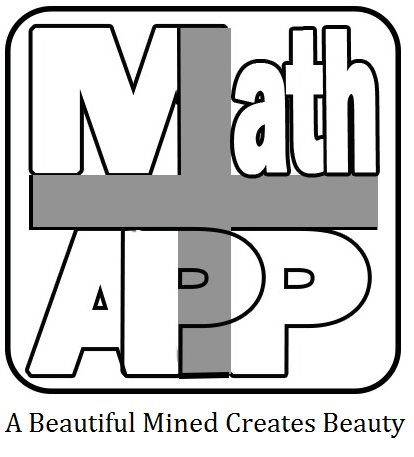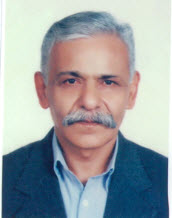## Prof. H. Doostie

doostih [AT] khu.ac.irدانشکده علوم ریاضی و کامپیوتر. شماره تماس : 34579600(026) و 77630040(021)

پست الکترونیکی:   doostih [AT] khu.ac.ir

حسین دوستی (بازنشسته) توضیحات استاد

1.    (Retired) Department of Mathematics, Kharazmi University, 49 Mofateh Ave., Tehran , Iran.

2.   (Honorary staff) Department of Mathematics, Science and Research Branch, Islamic Azad University, Tehran 14515/1775, Iran.

Phone: +98-21-77507772,   +98-26-34579600,    +98-21-44865015

Emails: doostih[AT]gmail.com,   doostih[AT]khu.ac.ir, doostieh[AT]yahoo.com

### EDUCATIONS

Ph.D.: St. Andrews University, Scotland, U.K. (1988).
M.Sc.: Mosahab Institute of Mathematics, Kharazmi University, Tehran, Iran (1975).
B.Sc.: Tabriz University, Iran (1973).

RESPONSIBILITIES

Head of Mathematics Department (Kharazmi University) during the years 1992-1994 and 2002-2004.

RESEARCH INTERESTS

Algebra (particularly: Combinatorial aspects in groups and semigroups)

TEACHING COURSES

B.Sc. courses:  Linear algebra, Groups and Rings, Graph theory, Differential equations.
M.Sc. courses: Finite mathematics, Finite groups.
Ph.D. courses:   Combinatorial group theory, Linear groups, Combinatorics, Lie Algebras.

PUBLICATIONS

1. RESEARCH ARTICLES
2. BOOKS (In Persian)
3. SUPERVISING (Ph.D. and M.Sc. Students)

1. RESEARCH ARTICLES

      C. M. Campbell, H. Doostie and E. F. Robertson, Fibonacci length of generating pairs in groups, In: Application of Fibonacci Numbers   3, 27-35, 1990.
      H. Doostie, The interesting classes of finite groups since 1976, Proc.  Of   22nd   Annual Iranian Mathematics Conference (Unive. of Ferdowsi Mashhad)  122-126,  1991.
      H. Doostie,   Non-metabelian  soluble  groups  involving  the  Lucas numbers,  J.  Sci.  Iran   3(1-2),  63-67,  1992.
         H. Doostie   and A. R. Jamali,  A  class of deficiency zero soluble groups of derived length 4,  Proc. Royal Soc.  Edinburgh, 121A,    163-168,  1992.
      H. Doostie, On the proof of a conjecture, Proc. of 24th Annual Iranian Mathematics Conference, ( Univ. of Shahid Beheshti), 112-117, 1993.
       H. Doostie, Generalized Fibonacci length, Proc. Of 27th Annual Iranians Mathematics Conference, 1996.
      H. Doostie, Two classes of one-relator product of  semigroups,  Proc. of 28th Annual  Iranian Mathematics Conference, (Tabriz Univ.), 153-158, 1997.
      H. Doostie,   An Identitifaction property in semigroups, Proc. of   28th   Annual Iranians Mathematics Conference, (Tabriz Univ.), 213-219, 1997.
       H. Doostie and   M. Hashemi, Two questions on the symmetric properties of semigroups, Proc. of  30th Annual Iranian   Mathematics Conference, 1999.
     H. Doostie and C. M. Campbell, Fibonacci length of automorphism groups involving the Tribonacci   numbers,   Vietnam  J. of  Math.  28,   57-65, 2000.
      H. Doostie and R. Golami, Computing on the Fibonacci length of finite groups, Internat.  J.  Appl. Math.  4(2) ,   149-156, 2000.
      C.M. Campbell, P.P. Campbell, H. Doostie and E.F. Robertson, On the Fibonacci length of powers of dihedral groups, In:  Application of Fibonacci Numbers  9, 69-75,  2000.
      H. Doostie , R.Golami and R.M. Thomas, Certain extensions of (l,m,n,k)-groups, Southeast Asian Bull. of  Math. 27( 1),  21-34,  2003.
      C.M. Campbell,  P.P. Campbell, H. Doostie and E.F. Robertson,  Fibonacci length for certain metacyclic groups, Algebra Colloquium 11(2),   215-229,  2004.
      H. Doostie  and M. Maghasedi,   Fibonacci length of direct product of groups, Vietnam J. of Math. 33(2), 189-197,  2005.
     H. Doostie and M. Maghasedi,    On the Fibonacci length of symmetric groups,  Internat. Rev. Pure   Appl. Math.  1(2),  155-163,  2005.
      H. Doostie and M. Hashemi, On the n-permutation property in semigroups and categories, Fareast J. of  Mathematical Sciences  19(2),  2005.
     H. Doostie and M. Maghasedi, On the group Aut(Sn), Proc. 36th Annual Iranians Mathematics Conference, 2005.
       H. Doostie and M. Hashemi, Fibonacci lengths involving the Wall number k(n), J. Appl. Math. and  Computing  20(1-2), 171-180, 2006.
      H. Doostie and L. Pourfaraj, On the minimal ideals of commuting regular rings and semigroups, Internat. J. of  Appl. Mathematics 19(2), 201-216,  2006.
      H. Doostie and P.P.Campbell, On the commutator lengths of finitely presented groups, Internat. J. of  Mathematics and Mathematical Sciences (IJMMS), Vol. 2006
      H. Doostie  and A.T. Adnani, Fibonacci length of certain nilpotent 2-groups, Acta Mathematica  Sinica 23( 5),  879-884,  2007.
       H. Doostie  and M. Hashemi, An application of the Fibonacci length, Internat. Mathematical  Forum  2(27),  ?-?,  2007.
       M. Hashemi and H. Doostie, An application of the Fibonacci lengths on graphs, Korean Annals of Math.  24,  49-56, 2007.
       H. Doostie  and L. Pourfaraj, Finite rings and Loop rings involving the commuting regular elements, Internat. Mathematical Forum 2(52),  2579-2586, 2007.
       M. Azadi, H. Doostie  and L. Pourfaraj, Certain rings and semigroups examining the regularity property, J.  Mathematics, Statistics and Alleid Fields  2(1), 1-6, 2008.
       H. Doostie and M. Maghasedi, Certain classes of groups with commutativity degree Ars  Combinatoria  89, 263-270, 2008.
       A. Sadeghieh and H. Doostie, The n-th roots of elements in finite groups, Mathematical Sciences (Quarterly Journal) 2(4), 347-356, 2008.
       K. Ahmadidelir, C.M. Campbell and H. Doostie, Two classes of finite semigroups and monoids involving Lucas numbers, Semigroup Forum 78(2), 200-209,  2009.
       H. Doostie and A. Sadeghieh, On the identification of subsemigroups of Transformation semigrous, J. of Mathematical Sciences: Advances& Applications 2(2), 245-256, 2009.
       H. Doostie ,  and K. Ahmadidelir, A class of Z-metacyclic groups involving Lucas numbers,  Novi-Sad  J.  of  Math.  39(1),  21-29,  2009.
       A. Sadeghieh and H. Doostie, Fibonacci length and the nth-root elements of Hamiltonian  groups, Internat. Mathematical Forum   4:39,  1923-1938, 2009.
      A. Sadeghieh and H. Doostie, Non-abelian sequenceable groups involving a-covers, J. of Sciences I.R.Iran  20(3),  277-282, 2009.
      H. Abdolzadeh, M. Azadi and H. Doostie, A subgroup involvement of Fibonacci length, J.  of   Appl. Math.  And   Computings 32, 382-392, 2010.
     A. Arjomandfar and H. Doostie, Proving the efficiency of pro-2-groups of fixed coclasses,  Bull.  Iranian Math. Soc.  37(4),   73-80,   2011.
      K. Ahmadidelir, C.M.Campbell and H. Doostie, Almost commutative semigroups, Algebra Colloquium  13(spec. 01), 881-888,    2012.
     A.  Arjomandfar,   C.M.  Campbell and H. Doostie,   Two classes of finite non-abelian p-sums semigroups,   Semigroup   Forum   85,   533-539,  2012.
     K. Ahmadidelir and H. Doostie,   On the automorphisms of direct product of monogenic  semigroups and monoids,  Turk. J.  Math. 36,   95-99, 2012.
     Bahram Ahmadi  and H. Doostie,   Numerical results on finite p-groups  of exponent p^2, Internat. J. of Mathematical Modeling & Computations, 2(2), 111-120, 2012.
     R. Golami, H. Doostie and and K. Ahmadidelir, Fibonacci length and special automorphisms of  finite (l, m | n, k)-groups.  TWMS J.  Pure   Appl. Math. 3(1),   182-189, 2012.
     N. Hosseinzadeh  and H. Doostie,  Presentation of semidirect product of monogenic semigroups, Internat.  Math. Forum  8(31), 1531-1537, 2013.
     Bahram Ahmadi and H. Doostie,  On a class of No-where commutative semigroup rings, Internat.  Math. Forum , 8(18),  859-863, 2013.
     Bahram Ahmadi and H. Doostie, On the 2-generator p-groups with non-cyclic commutator subgroups,  Azerbaijan  J. of  Math. 4(1),  73-78,  2014.
     Bahram Ahmadi,  C.M.  Campbell and H. Doostie,  Non-commutative finite monoids of a given order n>3,  An. St. Univ. Ovdius Constanta 22(2),  29-35,  2014.
     A. Gharibkhajeh and H. Doostie, A graphical difference between the inverse and regular semigroups,  Bull. Iranian Math. Soc.  40(2),  413-421,  2014.
     A. Gharibkhajeh and H. Doostie, On the graphs related to Green relations of finite semigroups,  Iranian J. Math. Sci.  and  Informatic 9(1), 42-50, 2014.
     M.R. Sorouhesh  and H. Doostie,  Quasi-commutative semigroups of finite order related to Hamiltonian groups, Bull. Korean  Math. Soc.  52(1), 239-246, 2015.
     A.  Firuzkuhy and H. Doostie, Commuting regularity of finite semigroups, Creative Math. and  Informatics 24(1), 43-47, 2015.
     B. Panbehkar and H. Doostie, More on the properties of union of two semigroups, Kasmera 43(1), 244-251, 2015.
     B. Panbehkar  and H. Doostie, Automatic semigroups producing an automaton semigroup, Southeast Asian Bull. of  Math. ??, 1-9, 2016.
     A.R. Pouhassani,  H. Doostie and H. Rasouli, Some elementary combinatorial properties of Aktas soft groups with contributions in soft actions, J. Informatics and Mathematical Sci. 8(3), 189-200, 2016.
     A.  Firuzkuhy and H. Doostie, Commuting regular graphs for non-commutative semigroups, Communication  in Math. and   Applications 7(2), 115-119, 2016.
  N. Hosseinzadeh and H. Doostie, Examples of non-quasi commutative semigroups decomposed into the union of groups, Bull. Iranian Math. Soc.  42(2),  483-487, 2016.
     M.R. Sorouhesh,  H. Doostie  and  C.M.  Campbell, A sufficient condition for coinciding the Green graphs of semigroups, J. Math. and Computer Sci. 17,   216-219,  2017.
     A.  Firuzkuhy and H. Doostie, Semigroups with maximum commuting regularity degree, JP  J. of Algebra, Number Theory and Applications 39(1), 77-86, 2017.
     M.M. Shamivand,  H. Doostie and H. Rasouli, Characterization of (m,n)-high-ideals of posemigroups when they are 0*-minimal, Communication in Math. And Applications 8(1), ??, 2017.
     B. Panbehkar  and H. Doostie, An automatic semigroup  of  languages, AAECC 28,  225-235,  2017.

1. BOOKS (In Persian)

 معادلات ديفرانسيل معمولي ترجمه انتشارات علوی 1362 مبانی کامپیوتر شیمی تاليف دانشگاه پیام نور 1365 جبر بروش تمرین جلد 1-2-3 ترجمه انتشارات مبتکران 1371 مبانی ریاضی تاليف انتشارات دانشگاه خوارزمی 1375 مبانی و کاربرد کامپیوتر در شیمی تاليف انتشارات مبتکران 1376 مبانی ریاضی به روش تمرین تاليف انتشارات مبتکران 1376 جبر دانشگاهی تاليف انتشارات مبتکران 1379 نظریه ی گراف و کاربردهای آن تاليف انتشارات مبتکران 1384 جبر بروش تمرین جلد 4-5-6 ترجمه انتشارات مبتکران 1384 مقدمه ای بر معادلات دیفرانسیل تاليف انتشارات روشنگران 1386
1. SUPERVISING (Ph.D. and MSc. Students)

3.1. Ph.D. Thesis

1. 1. Generalization of (l,m|n,k)-groups and their applications. Science and Research Branch, Islamic Azad University, Tehran, Iran, (2001), (Mr. Rahmat Golami).
2.  Numerical computations in finite groups. Science and Research Branch, Islamic Azad University, Tehran, Iran, (2005), (Mr. Mohammad Maghasedi).
3.  Fibonacci lengths of finite nilpotent groups.  Science and Research Branch, Islamic Azad University, Tehran,   Iran. (2005), (Mr. Amir Tabatabaie Adnani).
4.  Certain combinatorial results on the algebraic structures. Kharazmi University, Tehran, Iran, (2005), (Mr. Mansour Hashemi).
5.  On the minimal ideals of commuting regular rings and semigroups. Science and Research Branch, Islamic Azad University, Tehran, Iran, (2007), (Mr. Lotfolah Pourfaraj).
6.  Computing in p-groups. Science and Research Branch, Islamic Azad University, Tehran, Iran, (2008), (Mr. Mehrdad Azadi).
7.  On the order of elements in algebraic structures. Science and Research Branch, Islamic Azad University, Tehran, Iran, (2009), (Mr. Karim Ahmadidelir).
8.  Probabilistic aspects on the algebraic structures. Science and Research Branch, Islamic Azad University, Tehran, Iran, (2009), (Mr. Ali Sadeghieh).
9. Presentations of finite 2-groups.  Kharazmi University, Tehran, Iran, (2010), (Mr. Hossein Abdolzadeh).
10.  3-generated and 3-related groups and semigroups. Science and Research Branch, Islamic  Azad University, Tehran, Iran, (2010), (Mr. Abbas Arjomandfar).
11. On the non-linear irreducible characters. Kharazmi University, Tehran, Iran, (2012), (Mr. Amin Saeidi).
12. Green graphs in semigroups and Rank-two geometry graphs in non-abelian groups.  Science and Research Branch, Islamic Azad University, Tehran, Iran, (2013), (Mr. Amir Gharibkhajeh).
13. Finite p-groups of nilpotency class 3 and the related algebraic structures. Science and Research Branch, Islamic Azad University, Tehran, Iran, (2013), (Mr. Bahram Ahmadi).
14. Propertie of characters and representations of finite algebraic structures (semigroups and rings), Science and Research Branch, Islamic Azad University, Tehran, Iran, (2014), (Ms. Nargess Hosseinzadeh).
15. On the solvability and supersolvability of Lie algebras structures. Science and Research Branch, Islamic Azad University, Tehran, Iran, (2014), (Ms. Leila Goodarzi).
16. Quasi-commutative semigroups of finite order related to Hamiltonian groups. Science and Research Branch, Islamic Azad University, Tehran, Iran, (2014), (Mr. Mohammad Reza Sorouhesh).
17. Commuting regularity degree in Algebraic structures.  Science and Research Branch, Islamic Azad University, Tehran, Iran, (2016), (Mrs. Azam Firuzkuhy).
18. Automata and algebraic structures. Science and Research Branch, Islamic Azad University, Tehran, Iran, (2016), (Mr. Bozorgh Panbehkar).
19. Investigating semigroups and non-commutative semirings. Science and Research Branch, Islamic Azad University, Tehran, Iran, (2016), (Mr. Mohammad Mehdi Shamivand).
20. Study of algebraic and categorical properties of LA-acts over LA-semigroups.  Science and Research Branch, Islamic Azad University, Tehran, Iran, (2016), (Mr. Alireza Shaabani).
21. Algebraic structures on soft sets (groups and graphs). Science and Research Branch, Islamic Azad University, Tehran, Iran, (2017), (Mr. Alireza Pouhassani).

3.2.  M.Sc. Dissertations

1. Classes of deficiency zero groups related to (2,n)-groups. Kharazmi University, Tehran, Iran. (1990),  (Mr. Jamal Saffar Ardabili).
2. Symmetrically presented groups. North Branch Islamic Azad University, Tehran, Iran. (1992),   (Mr. Azim Niknehad).
3.  On a conjecture about the deficiency zero groups F^{a,b,c}. Kharazmi University, Tehran, Iran. (1992), (Mr. Morteza Gorzinnejad).
4. Deficiency zero groups involving the Lucas numbers. North Branch Islamic Azad University, Tehran, Iran. (1993), (Mr. Shahyar Pakdaman).
5. An Algorithm for |G’/G”|. North Branch Islamic Azad University, Tehran, Iran. (1993), (Mr. Shahram Najafzadeh).
6.  Derivation and integration in the free group-rings. North Branch Islamic Azad University, Tehran, Iran. (1993), (Mr. Ghasem Teymouri).
7. The Schur Multiplier. Kharazmi University, Tehran, Iran. (1993), (Mr. Mahmoud Karimi-e-Hosseini).
8. The Fibonacci length of metacyclic groups. Kharazmi University, Tehran, Iran. (1994), (Mr. Hamid Bagheri).
9. A class of symmetrically presented groups. Kharazmi University, Tehran, Iran. (1997), (Mr. Abbas Moeeni-e-Rad).
10. Automorphisms of a finite group. Kharazmi University, Tehran, Iran. (1997), (Mehrdad Akbarin).
11. Presentation of groups and semigroups. Kharazmi University, Tehran, Iran. (1997), (Mr. Jamal-addin Hosseini).
12.  One relator and semi-direct products of semigroups. Kharazmi University, Tehran, Iran. (1998), (Mr. Seyed Mohammad Tavalla).
13. Nilpotency class of stability group. Kharazmi University, Tehran, Iran. (2000), (Mr. Mohammad Hossein Salmani).
14. Counting the finite groups. Shahid Rajaee University, Tehran, Iran. (2001), (Mr. Nasir Haghshenas).